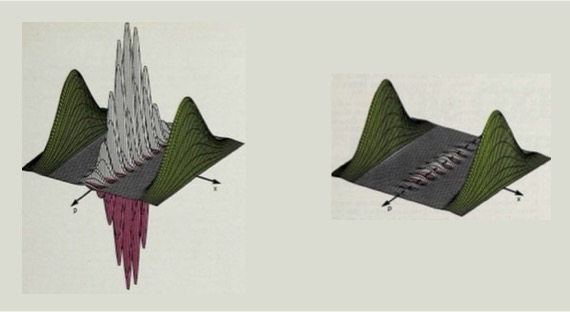# Open Quantum Systems# Department of Physics and Astronomy

##### Teaching Assistants: Andrew Forbes & S. Vikas Buchemmavari

Office Hours: TBA

Synopsis: All quantum systems interact with their environment, be it through unwanted interactions with a noisy bath of thermal and quantum fluctuations, or because we want to observed the system through a quantum measurement.  This class will cover the foundations of open quantum systems and its relationship to classical nonequilibrium statistical physics.  New features arise in quantum mechanics  -- entanglement and negative probabilities -- with profound implications.   With a foundation in open quantum systems, we will address fundamental processes in quantum mechanics: dissipation, decoherence, and measurement.

General Information

"Recommended" Texts (none required)
• The Theory of Open Quantum Systems, by H.-P. Breuer and F. Petruccione
• Quantum Noise: A Handbook of Markovian and Non-Markovian Quantum Stochastic Methods with Applications to Quantum Optics, by C. Gardiner and P. Zoller
• Handbook of Stochastic Methods: For Physics, Chemistry, and the Natural Sciences, by C. Gardiner

* Problem Sets (5-8 assignments) 75%
* Final Project 25%
*Problem sets will be available on the web, about every other week. Generally assignments will be due Thursdays in the TA mailbox.

### Tentative Syllabus

#### I. Quantum Mechanical Building Blocks

- Closed quantum systems: states and evolution
- Two level systems:  qubits
- Infinite dimension systems: bosons
- Multipartite systems: entanglement

#### II. Foundation theory of open quantum systems

- Completely positive maps and quantum channels
- Kraus representation
- Quantum channels for qubits
- Measurment model

#### III. Lindblad Master Equation - formal theory

- Markov differential maps - Lindblad form
- Born-Markov approximation and the derivation of the Master Equation
- Examples for qubits: amplitude damping, dephasing, depolarizating channels
- Damped simple harmonic oscillator

#### IV. Langevin equations

- Stochastics processes
- Brownian motion
- Langevin equation
- Heisenberg-Langevin

#### V. Fokker-Planck equation

- Classical statistical physics theory
- Damped harmonic oscillator
- Decoherence and the emergence of the classical world

#### VI. Quantum Trajectories

- Quantum trajectories — the measurement model
- Quantum Monte-Carlo wavefunctions
- Different unravellings of master equation
- Stochastic Schrödinger equation and continuous measurement

 Jan. 17 Introduction to Open Quantum Systems: Dissipation, Irreversibility,  Decoherence, Measurement Lecture #1 Jan. 19 Quantum Foundations: Density operators, Measurements qubits,  Bloch sphere Lecture #2 Jan. 24 Qubit Dynamics: Rabi Oscillations Lecture #3 Jan. 26 Qubits : Phenomenological Damping - Bloch equations Lecture #4 Jan. 31 Continuous Variable Quantum Mechanics:  Simple harmonic oscillator, coherent states, boson algebra Lecture #5 Feb.2 Introduction to Phase Space Representations Lecture #6 Feb. 7 Continuation Feb. 9 Quasiprobability functions Wigner (W), Husumi (Q), and Glauber (P) Feb. 14 Dynamics in Phase Space Feb. 16 Tensor product structure and entanglement Schmidt decomposition Lecture #7 Feb. 21 Intro to open quantum system dynamics:  Quantum operations, CP maps, Kraus Representation Lecture #8 Feb. 23 Quantum Channels for qubits Feb. 28 Irreversible bipartite system-reservoir interaction. Markov approximation - Lindblad Master Equation Lecture #9 Mar. 2 Derivation of the Lindblad Master Equation Born-Markov approximation Mar. 7 Examples of Master Equation Evolution: Damped two-level atom Lecture #10 Mar. 9 Damped Simple Harmonic Oscillator Mar. 13-17 Spring Break Mar. 21 No Class Mar. 23 Damped Simple Harmonic Oscillator in Phase Space Lecture #11 Mar. 28 Decoherence and the Emergence of the Classical World Mar. 30 Continuation Apr. 4 Brownian Motion and Heisenberg-Langevin Equation Lecture #12 Apr. 6 No lecture Apr. 11 Continuation Apr. 13 Classical stochastic processes and the master equation Lecture #13 Aprl. 18 Wiener processes and Ito stochastic differential equations Apr. 20 Continuation Apr. 25 Quantum Trajectories I Measurement model Lecture #14 Molmer 1 Molmer 2 Apr. 27 Quantum Trajectories II Quantum Monte-Carlo Wave Function Algorithm Lecture #15 Molmer 3 Molmer 4 May 2 Quantum Trajectories III Different Unravelings of the Master Equation Lecture #16 May 4 The Stochastic Schrodinger Equation. Quantum State Diffusion Lecture #17 May 9 Continuous Measurement Lecture #18

Problem Sets

 Problem Set #1 Problem Set #2 Problem Set #3 Problem Set #4 Problem Set #5 Final Project